IF YOU CAN SEE THIS go to /sfa/Nuprl/Shared/Xindentation_hack_doc.html

### Types versus Propositions

Constructive type theorists attempt to account for certain key features of propositions. The basic type theoretic claim is that the "computational content" of a proposition P can be expressed as a type T, this content being what is available to the procedure that is implicit, according to constructivists, in a proof of any implication "P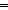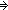?".

It should be noted that the operators we have been displaying as the logical operators are actually defined as type-valued functions. See Propositions as Types - standard defs. The connection between the usual logical operators and these type operators requires explanation.

We shall focus on a few aspects of constructive mathematical language: effective notations, computational content, and existential proof witnesses.

Effective Notation

The core of computational type theory is a semantic method for notating values and types that is intended to be adequate for constructivist mathematical expression. See Types.

A paradigm for constructively adequate notation is notating natural numbers. From a(n adequate) notation for a number, one can effectively determine whether it denotes zero, and if it does not, then one can effectively derive a notation for its predecessor. Similarly, from a notation for an ordered pair one must be able to derive a notation for each component.
Unless otherwise stipulated, we'll use the word "notation" to refer to an effective notation in this sense.

Notations for functions inherit criteria for effectiveness from their output types, and can exploit effectiveness of notations for their arguments. A notation for a (one-place) function is, at least in part, a way of building a notation for its result for any argument from a notation for that argument. So, from notations for a natural-number-valued function and for an argument, one can build a notation for the function's value and ascertain whether it denotes zero, et cetera.

Computational Content and Constructive Choice

Constructivists maintain that constructively understanding what a proposition means requires a conception of "computational content" of possible proofs of that proposition. Further, if one understands a proof then one can come up with its computational content. In particular, the computational content of a proof of an implication "PQ" is taken to be an effective method for deriving from the computational content of any proof of P the computational content of a proof of Q. (It should be noted that there is no general acceptance among constructivists that Church's thesis is correct with regard to the identity between effective procedures and recursive enumerability, even when restricted to numeric functions.)

Similarly, the computational content of a proof that "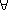x:AQ(x)" is taken to be a method which from any effective notation "r" referring to a member of type A, together with any further computational content there may be in a proof that r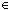A, will construct the computational content of a proof of the proposition Q(r). Constructivists typically also presuppose that these methods are deterministic, making this interpretation of universal quantification a sort of choice principal.

Constructive type theory claims that the computational content of all the possible proofs of a proposition constitutes a type, and that giving a complete explanation of the meaning of a proposition requires explaining what that corresponding type of computational content is. Thus, the type of computational content for proofs of "PQ" is "A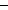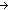B" if A and B are the types of computational content for proofs of P and of Q. The computational type theory of Nuprl has no explicit logic of propositions, and is really just a logic of functions and types. The interpretation of types as the propositions whose computational content they describe is an informal one, albeit one encouraged by the practice of adopting standard propositional notations but defining them as type notations. See Propositions as Types - standard defs.

Existential Proof Witnesses

From a (constructive) proof of an existential proposition "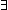x:AP(x)" one can find a notation "r" referring to a member of A, and can also produce a proof of "P(r)".
Consequently, for example, since Thm* P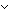Q(x: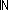. (x = 0P) & (x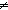0Q)), then from any proof of "PQ" one can produce a proof of "P" or a proof of "Q". Here's how.
Use modus ponens on the above theorem to get a proof of "x:. (x = 0P) & (x0Q)" and find a witness rand a proof of "(r = 0P) & (r0Q)". Decide whether "r" denotes zero and if so use modus ponens on "r = 0P" to get a proof of "P"; otherwise, use modus ponens on "r0Q" to get a proof of "Q".

This example leads to the famous intuitionist rejection of the "law of the excluded middle",A:Prop. A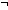A. It is quite implausible that one could always effectively determine whether an arbitrary proposition were true. The above argument depends critically on the requirement that the intermediate existential witness be an effective notation for a number. In a non-constructive language one might simply use the trivial witness expression "if P is true then 0, otherwise 1", but this would not count as an effective notation unless one already had a way of determining "P" as true or as false.

Thus, constructively, one may not always perform a case split on whether a proposition is true. One may do so for a proposition P when "PP", which we abbreviate by Def Dec(P) == PP.

Thm* Dec(P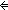(b: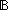Pb) [there is a coercion fromto Prop]

It should be noted that we do not mean by "proof" here a particular formalization of proof, such as Nuprl Proofs, but the full informal notion of constructive proof, which is conceived of as open-ended.

(Sept 2003 - sfa )